# Factorial - math word problems

#### Number of problems found: 51

• Big factorial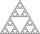How many zeros end number 116! ?
• Cancel fractionsCompress the expression of factorial: (n+6)!/(n+4)!-n!/(n-2)!
• Value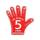Find the value of the expression: 6!·10^-3
• Possible combinations - word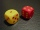How many ways can the letters F, A, I, R be arranged?
• Committees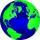How many different committees of 2 people can be formed from a class of 21 students?
• Permutations without repetitionFrom how many elements, we can create 720 permutations without repetition?
• WordWhat is the probability that a random word composed of chars E, Y, G, E, R, O, M, T will be the GEOMETRY?
• Playing cards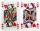How many possible ways are to shuffle 7 playing cards?
• Vans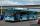In how many ways can 9 shuttle vans line up at the airport?How many ways can we thread 4 red, 5 blue, and 6 yellow beads onto a thread?
• Practice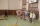How many ways can you place 20 pupils in a row when starting on practice?
• Shelf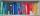How many ways are there to arrange 6 books on a shelf?
• Tournament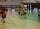Determine how many ways can be chosen two representatives from 34 students to school tournament.
• Bookshelf and books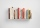How many ways can we place 7 books in a bookshelf?
• PrizeHow many ways can be rewarded 9 participants with the first, second and third prize in a sports competition?
• Seating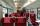How many ways can 6 people sit on 6 numbered chairs (e. g. , seat reservation on the train)?
• Guests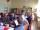How many ways can 9 guests sit down on 10 seats standing in a row?
• Elections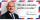In elections candidate 10 political parties. Calculate how many possible ways can the elections finish, if any two parties will not get the same number of votes.
• Elements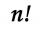If the number of elements is decreased by two the number of permutations is decreased 30 times. How many elements are?
• Olympics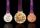In how many ways can be placed 6 athletes on the podium at the Olympics? Depend on the color of the metal.

Do you have an interesting mathematical word problem that you can't solve it? Submit a math problem, and we can try to solve it.

We will send a solution to your e-mail address. Solved examples are also published here. Please enter the e-mail correctly and check whether you don't have a full mailbox.

Please do not submit problems from current active competitions such as Mathematical Olympiad, correspondence seminars etc...